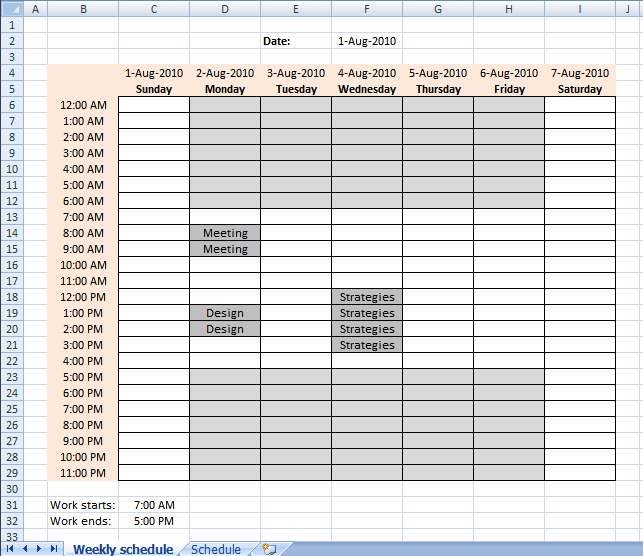Author: Oscar Cronquist Article last updated on December 28, 2018The image above demonstrates conditional formatting highlighting hours outside work hours, those cells are filled with grey except weekends.

Conditional formatting formula applied on cell range C6:I29:

=AND(OR(\$B6<\$C\$31, \$B6>=\$C\$32), AND(WEEKDAY(C\$4)<7, WEEKDAY(C\$4)>1))

This formula checks if the weekday in C4:I4 is 2,3,4,5 or 6. (Monday to Friday) and if the time in cell range B6:B29 is outside workhours specified in cell C31 and C32. If formula returns TRUE, the cell is filled grey.

### Explaining conditional formatting in cell C9

#### Step 1 - Check if time \$B6 is less than start hour \$C\$31

\$B6<\$C\$31

becomes

0<0.291666666666667

and returns TRUE.

#### Step 2 - Check if time \$B6 is larger than or equal to start hour \$C\$32

\$B6>=\$C\$32

becomes

0>=0.708333333333333

and returns FALSE

#### Step 3 - If any of the logical expressions evaluate to TRUE then return TRUE

The OR function checks whether any of the arguments are TRUE or FALSE and returns FALSE if  all arguments are FALSE.

OR(\$B6<\$C\$31, \$B6>=\$C\$32)

becomes

OR(TRUE, FALSE)

and returns TRUE.

#### Step 4 - Check if weekday is less than 7 (Saturday)

The WEEKDAY function returns a number from 1 to 7 identifying the day of the week of a date.

WEEKDAY(C\$4)<7

becomes

WEEKDAY(40391)<7

and returns TRUE.

#### Step 5 - Check if weekday number is larger than 1 (Sunday)

WEEKDAY(C\$4)>1

becomes

WEEKDAY(40391)<1

and returns FALSE.

#### Step 6 - Both logical expressions must be TRUE

The AND function checks whether all arguments are TRUE and returns TRUE if all arguments are TRUE.

AND(WEEKDAY(C\$4)<7, WEEKDAY(C\$4)>1)

becomes

AND(TRUE, FALSE)

and returns FALSE.

#### Step 7 - AND logic

AND(OR(\$B6<\$C\$31, \$B6>=\$C\$32), AND(WEEKDAY(C\$4)<7, WEEKDAY(C\$4)>1))

becomes

AND(TRUE, FALSE)

and returns FALSE. Cell C6 is not highlighted.

### Get the Excel fileHighlight-work-hours-in-a-weekly-schedule.xlsx